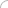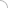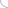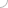# A+ and Basic PC interview questions and answers

## A+ and Basic PC interview questions and answers

### Q1. What type of programming language is A+? Q2. What was the reason behind creating the A+ language? Q3. What were the changes that the A+ language brought about in APL? Q4. What is the development tool that is used for A+? Q5. What is the primary usage of the A+ language? Q6. Can A+ be run under Windows? Q7. What are the highlights of A+? Q8. What is the rank operator? Q9. Define a slotfiller in reference to A+? Q10. State the precedence rules of A+? Q11. Define monadic and dyadic functions, give examples? Q12. What type of control statements are used in A+? Q13. How can monadic functions be categorized? Q14. What is Absolute value |x , provide an example? Q15. How can dyadic functions be categorized? Q16. Define Equal to y=x function with the help of an example? Q17. How are numbers represented in A+? Q18. What is the value at which the comparison tolerance is fixed at? Q19. What is an operator and define its syntax with the help of an example? Q20. What are dependencies and how are they established in A+? Q21. What are data files in A+ known as and why? Q22. Define any two display classes used in A+? Q23. What is acronym of BASIC? Q24. What was the purpose of creating BASIC? Q25. How is basic different from other programming languages? Q26. What is the purpose of subroutines in reference to BASIC? Q27. How are numbers represented in BASIC? Q28. What are variables and how are they denoted in BASIC? Q29. Define expressions in basic with the help of an example? Q30. State the rules by which an expression is interpreted? Q31. How are loops implemented in BASIC, state with an example? Q32. What do the Int and Rnd functions perform in BASIC? Q33. What does the CLS command perform in BASIC? Q34. What does the END command in a BASIC program signify? Q35. What are BASIC dialects, give two examples? Q36. Define the meaning of time sharing systems in reference to BASIC? Q37. Write a program performing basic arithmetic operations using BASIC. Q38. What are the different conditional statement structures that can be used in BASIC? Q39. Differentiate between shared and local variables? Q40. What is recursion? Explain with an example in reference to BASIC? Q41. What is strong typing in reference to BASIC? Q42. Explain the CIRCLE command with syntax and an example. Q43. Explain the FOR-NEXT command with syntax and an example. Q44. Write a program in BASIC to create sounds. Q45. Show the use of the screen coordinates command with the help of an example. Q46. What are reserved words in reference to BASIC, give two examples. Q47. Explain the concept of structured programming in reference to BASIC? (adsbygoogle = window.adsbygoogle || []).push({}); Write your comment - Share Knowledge and Experience Discussion Board Regarding Answers I need the answers of those questions above.Plz email me - meenakshi.sodhi8@gmail.com Meenakshi 09-8-2016 04:00 AM Need Answer I need the answers of those questions above.Plz email me - shovon.1985@gmail.com shovon 10-4-2014 01:03 PM A+ pc engineering I want A+ pc engineering interviwe questions and answers plz send me mail to me abdulraheem 12-19-2012 05:44 AM   (adsbygoogle = window.adsbygoogle || []).push({}); (adsbygoogle = window.adsbygoogle || []).push({});Interview questionsLatest MCQs » General awareness - Banking » ASP.NET » PL/SQL » Mechanical Engineering » IAS Prelims GS » Java » Programming Language » Electrical Engineering » English » C++ » Software Engineering » Electronic Engineering » Quantitative Aptitude » Oracle » English » Finance Home | About us | Sitemap | Contact us | We are hiring © Copyright 2016. All Rights Reserved. Terms of use  |  Follow us on Facebook!